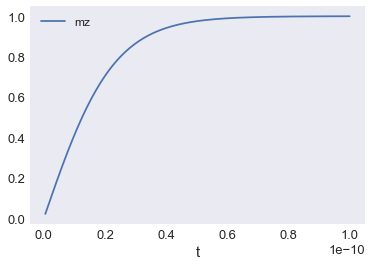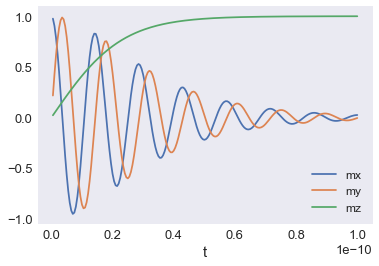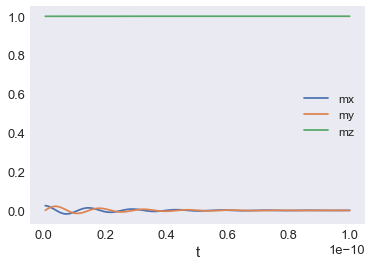# Getting started 03: Dynamics¶

Interactive online tutorial:The dynamics of magnetisation field $\mathbf{m}$ is governed by the Landau-Lifshitz-Gilbert (LLG) equation

$$\frac{d\mathbf{m}}{dt} = \underbrace{-\gamma_{0}(\mathbf{m} \times \mathbf{H}_\text{eff})}_\text{precession} + \underbrace{\alpha\left(\mathbf{m} \times \frac{d\mathbf{m}}{dt}\right)}_\text{damping},$$

where $\gamma_{0}$ is the gyromagnetic ratio, $\alpha$ is the Gilbert damping, and $\mathbf{H}_\text{eff}$ is the effective field. It consists of two terms: precession and damping. In this tutorial, we will explore some basic properties of this equation to understand how to define it in simulations.

We will study the simplest "zero-dimensional" case - macrospin. In the first step, after we import necessary modules (oommfc and discretisedfield), we create the mesh which consists of a single finite cell.

In :
import oommfc as oc
import discretisedfield as df
import micromagneticmodel as mm
%matplotlib inline

# Define a macrospin mesh (i.e. one discretisation cell).
p1 = (0, 0, 0)  # first point of the mesh domain (m)
p2 = (1e-9, 1e-9, 1e-9)  # second point of the mesh domain (m)
n = (1, 1, 1)  # discretisation cell size (m)

region = df.Region(p1=p1, p2=p2)
mesh = df.Mesh(region=region, n=n)


Now, we can create a micromagnetic system object.

In :
system = mm.System(name='macrospin')


Let us assume we have a simple Hamiltonian which consists of only Zeeman energy term

$$w = -\mu_{0}M_\text{s}\mathbf{m}\cdot\mathbf{H},$$

where $M_\text{s}$ is the saturation magnetisation, $\mu_{0}$ is the magnetic constant, and $\mathbf{H}$ is the external magnetic field. We apply the external magnetic field with magnitude $H = 2 \times 10^{6} \,\text{A}\,\text{m}^{-1}$ in the positive $z$ direction.

In :
H = (0, 0, 2e6)  # external magnetic field (A/m)
system.energy = mm.Zeeman(H=H)


In the next step we can define the system's dynamics. Let us assume we have $\gamma_{0} = 2.211 \times 10^{5} \,\text{m}\,\text{A}^{-1}\,\text{s}^{-1}$ and $\alpha=0.1$.

In :
gamma0 = 2.211e5  # gyromagnetic ratio (m/As)
alpha = 0.1  # Gilbert damping

system.dynamics = mm.Precession(gamma0=gamma0) + mm.Damping(alpha=alpha)


To check what is our dynamics equation:

In :
system.dynamics

Out:
$-\frac{\gamma_{0}}{1 + \alpha^{2}} \mathbf{m} \times \mathbf{H}_\text{eff}-\frac{\gamma_{0} \alpha}{1 + \alpha^{2}} \mathbf{m} \times (\mathbf{m} \times \mathbf{H}_\text{eff})$

Before we start running time evolution simulations, we need to initialise the magnetisation. In this case, our magnetisation is pointing in the positive $x$ direction with $M_\text{s} = 8 \times 10^{6} \,\text{A}\,\text{m}^{-1}$. The magnetisation is defined using Field class from the discretisedfield package we imported earlier.

In :
initial_m = (1, 0, 0)  # vector in x direction
Ms = 8e6  # magnetisation saturation (A/m)

system.m = df.Field(mesh, dim=3, value=initial_m, norm=Ms)


Now, we can run the time evolution using TimeDriver for $t=0.1 \,\text{ns}$ and save the magnetisation configuration in $n=200$ steps.

In :
td = oc.TimeDriver()
td.drive(system, t=0.1e-9, n=200)

Running OOMMF (ExeOOMMFRunner) [2020/06/14 11:16]... (3.7 s)


How different system parameters vary with time, we can inspect by showing the system's datatable.

In :
system.table.data

Out:
E E_calc_count max_dm/dt dE/dt delta_E E_zeeman iteration stage_iteration stage mx my mz last_time_step t
0 -4.400762e-22 37.0 25204.415522 -8.798712e-10 -3.269612e-22 -4.400762e-22 6.0 6.0 0.0 0.975901 0.217115 0.021888 3.715017e-13 5.000000e-13
1 -8.797309e-22 44.0 25186.311578 -8.786077e-10 -4.396547e-22 -8.797309e-22 8.0 1.0 1.0 0.904810 0.423562 0.043754 5.000000e-13 1.000000e-12
2 -1.318544e-21 51.0 25156.186455 -8.765071e-10 -4.388134e-22 -1.318544e-21 10.0 1.0 2.0 0.790286 0.609218 0.065579 5.000000e-13 1.500000e-12
3 -1.756100e-21 58.0 25114.112032 -8.735776e-10 -4.375555e-22 -1.756100e-21 12.0 1.0 3.0 0.638055 0.765021 0.087341 5.000000e-13 2.000000e-12
4 -2.191985e-21 65.0 25060.188355 -8.698302e-10 -4.358857e-22 -2.191985e-21 14.0 1.0 4.0 0.455710 0.883427 0.109020 5.000000e-13 2.500000e-12
... ... ... ... ... ... ... ... ... ... ... ... ... ... ...
195 -2.009865e-20 1402.0 690.438568 -6.602614e-13 -3.374608e-25 -2.009865e-20 396.0 1.0 195.0 0.013011 -0.024099 0.999625 5.000000e-13 9.800000e-11
196 -2.009897e-20 1409.0 675.493807 -6.319876e-13 -3.230101e-25 -2.009897e-20 398.0 1.0 196.0 0.017545 -0.020251 0.999641 5.000000e-13 9.850000e-11
197 -2.009928e-20 1416.0 660.872303 -6.049242e-13 -3.091780e-25 -2.009928e-20 400.0 1.0 197.0 0.021059 -0.015612 0.999656 5.000000e-13 9.900000e-11
198 -2.009958e-20 1423.0 646.567078 -5.790193e-13 -2.959381e-25 -2.009958e-20 402.0 1.0 198.0 0.023428 -0.010435 0.999671 5.000000e-13 9.950000e-11
199 -2.009986e-20 1430.0 632.571305 -5.542233e-13 -2.832649e-25 -2.009986e-20 404.0 1.0 199.0 0.024591 -0.004988 0.999685 5.000000e-13 1.000000e-10

200 rows × 14 columns

However, in our case it is much more informative if we plot the time evolution of magnetisation $z$ component $m_{z}(t)$.

In :
system.table.data.plot('t', 'mz');Similarly, we can plot all three magnetisation components

In :
system.table.data.plot('t', ['mx', 'my', 'mz']);We can see that after some time the macrospin aligns parallel to the external magnetic field in the $z$ direction.

### Exercise 1¶

By looking at the previous example, explore the magnetisation dynamics for $\alpha=0.005$ in the following code cell.

In :
# Uncomment and modify the value of Gilbert damping in the following line.
# system.dynamics.damping.alpha = 1
system.m = df.Field(mesh, dim=3, value=initial_m, norm=Ms)

td.drive(system, t=0.1e-9, n=200)

system.table.data.plot('t', ['mx', 'my', 'mz']);

Running OOMMF (ExeOOMMFRunner) [2020/06/14 11:17]... (3.7 s)### Exercise 2¶

Repeat the simulation with $\alpha=0.1$ and $\mathbf{H} = (0, 0, -2\times 10^{6})\,\text{A}\,\text{m}^{-1}$.

In :
# Uncomment and modify the value in the following line
# system.hamiltonian.zeeman.H = (0, 0, -2e6)
system.dynamics.damping.alpha = 0.1
system.m = df.Field(mesh, dim=3, value=initial_m, norm=Ms)

td.drive(system, t=0.1e-9, n=200)

system.table.data.plot('t', ['mx', 'my', 'mz']);

Running OOMMF (ExeOOMMFRunner) [2020/06/14 11:17]... (3.3 s)### Exercise 3¶

Keep using $\alpha=0.1$. Change the field from H = (0, 0, -2e6) to H = (0, -1.41e6, -1.41e6), and plot $m_x(t)$, $m_y(t)$ and $m_z(t)$ as above. Can you explain the (initially non-intuitive) output?

In :
# Uncomment and modify the value in the following line.
# system.hamiltonian.zeeman.H = (0, 0, 0)

td.drive(system, t=0.1e-9, n=200)

system.table.data.plot('t', ['mx', 'my', 'mz']);

Running OOMMF (ExeOOMMFRunner) [2020/06/14 11:17]... (3.7 s)